User’s Guide : Advanced Single Equation Analysis : Elastic Net and Lasso : Working with Elastic Net Equations

Working with Elastic Net Equations
The elastic net equation estimated by EViews is a penalized regression specification. As a result, most of the views you may be accustomed to for other linear and nonlinear regression equations are not available. Fortunately, we have supplied several additional views applicable to elastic net models and cross-validation. We describe below the basics of working with your elastic net equation, focusing on the views specific to this estimation method.
Estimation Output
Following estimation, EViews displays the elastic net, Lasso, or ridge output. For example, we might have: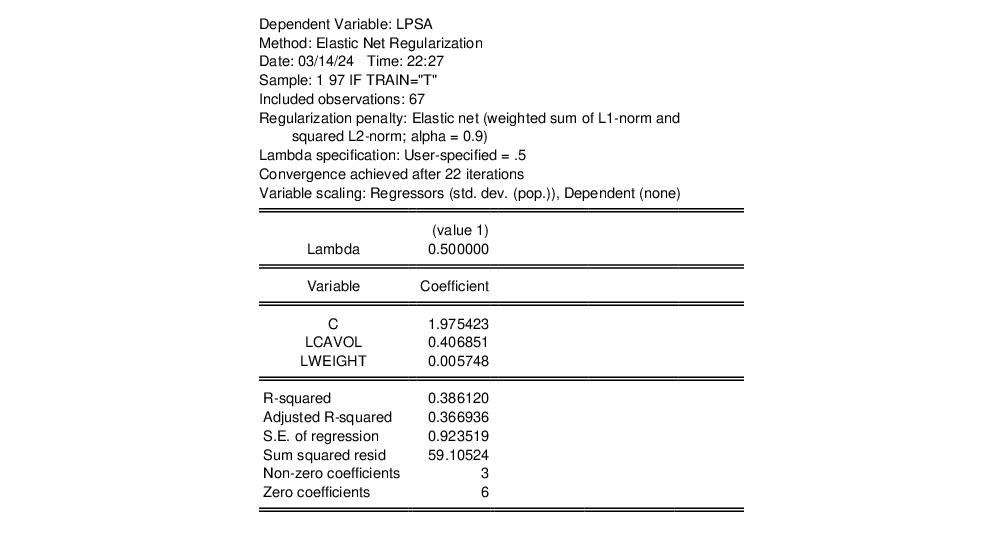The top portion of the output displays the dependent variable, method, date, sample information, description of the penalty type and alpha parameter, information about cross-validation if it has been used, the transformation of the regressors, and information about the estimation procedure. In this example, we used the default elastic net penalty with an alpha value of 0.5, midway between a ridge and Lasso model, as well as the default 5-fold cross-validation. Out of the list of lambda values used in the cross-validation procedure (available in the views, described below) EViews has chosen the value 0.04776 as having the lowest mean squared error. No transformation was done on the regressors.
The middle part of the output displays coefficient values for the certain values of lambda. If cross-validation has been done with multiple training and test sets, the first column supplies the coefficients associated with the minimum measurement error (associated with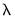), and the second and third columns give the coefficients for the largest values of the penalty parameter within one and two standard errors of the minimum error (associated with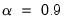and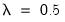). If one, two, or three values of lambda are provided, the output will simply display the coefficients for these values.
The bottom portion of the output is very different from what you may be used to in EViews. The first row contains the model degrees of freedom, the second is the L1 norm, and the third is the R-squared.
Elastic Net Views and Procs
There is little overlap between the views and procs for elastic net equations and many of the other estimators in EViews such as least squares. We will not discuss the handful of similar views, such as the residuals and derivatives views, but will focus instead on new views specific to elastic net, ridge, and Lasso estimators.
Representations View
As always, the representations view (View/Representations) shows the estimation command, estimation equation, and substituted coefficients sections.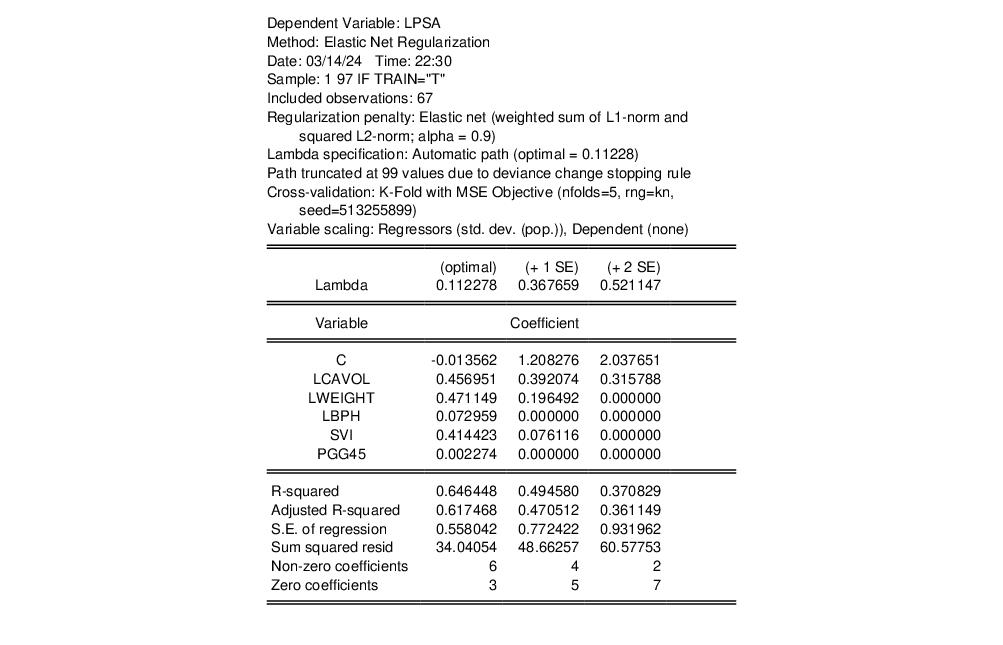Coefficient Matrix
The Coefficient Matrix view is one of two views that allow you to easily see the behavior of the model over the entire lambda path. The leftmost column lists all the lambdas used in the estimation. The remaining columns display the coefficients at each value of lambda.Summary Path
Select View/Summary Path to show the lambda path in the leftmost column, the model degrees of freedom in the second column, the L1 norm of the coefficients in the next column, and the R-squared of the model.Graphs
The Coefficient Graphs section of the View menu has options for displaying the evolution of the coefficients according to various features of the model.
Lambda Coefficient
To display a graph of the coefficients, ordered by the values of lambda in the lambda path, select View/Coefficient Graphs/Lambda:This is the graph you would see by plotting each column (excluding the constant, if included) of the View/Coefficient Matrix table against the leftmost lambda path column. In this example we see that as the value of the regularization penalty lambda increases, the absolute value of each of the coefficients decreases. This is as we would expect for our penalized regression. The thin black vertical line is the minimum value of lambda selected by cross-validation.
L1 Norm Coefficient
To display a graph of the coefficients against the L1 norm of the coefficients (again excluding the constant if included), select View/Coefficient Graphs/L1 Norm:In this graph we can see how the proportion of each coefficient in the norm changes as the norm of all the coefficients changes.
R-squared coefficient
The plot of the coefficients against the R-squared value of the coefficients is under View/Coefficient Graphs/R-squared:We can see in this example that the R-squared statistic is larger where the absolute values of the coefficients are larger.
Diagnostics
If cross-validation has been done EViews provides diagnostic tools for evaluating the results. These consist of a graph and table of the errors from the training and test sets, graphs of the evolution of the objective function for each value of lambda, and tables of the indices for the training and test sets.
Training/Test Error Graph
To display a graph of the errors produced by cross-validation, choose View/Diagnostics/Training/Test Error Graph:In this graph we see the lambda path on the x-axis and the error measure means of the training and test sets on the y-axis. As we would expect, the training error is consistently smaller than the test error. This graph is what we see by plotting the first three columns of the Training/Test Error Table, below.
Training/Test Error Table
Under View/Diagnostics/Training/Test Error Table you can view a table containing the information in the Training/Test Error Graph graph.The first column contains the lambda path, the second and third columns list the means over the test and training sets from the cross-validation, and the fourth and fifth columns are the associated standard errors. For example, 5-fold cross-validation performed on a dataset of 100 values divides the data into five sets of twenty data points. For each division of training and test sets, EViews calculates an error measure over the residuals. When repeated over each training/test set, we are left with a series of five error measures that we then use for the mean and standard error.
Train/Test Set Indices
In View/Diagnostics/Training/Training Sets Table and Test Sets Table you can see tables of the data indices selected by cross-validation for the training and test sets.At the top of the Training Sets Table are the indices of each cross-validation set (for example, 1 through 5 for 5-fold cross-validation). Down each column are the indices of the data points selected for each cross-validation training set.
Forecasting
Static and dynamic forecasting from an elastic net equation is as straightforward as forecasting from a least squares equation object. To perform the forecast simulation, click on the Forecast button on the equation toolbar or select Proc/Forecast... from the equation menu to display the dialog.